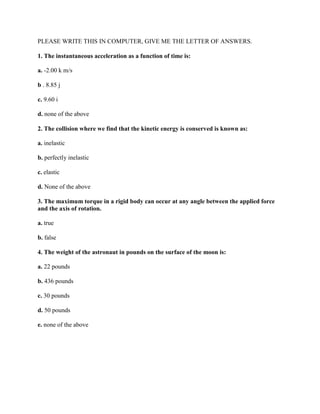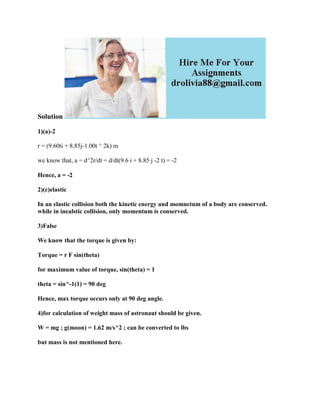Diese Präsentation wurde erfolgreich gemeldet.

# PLEASE WRITE THIS IN COMPUTER- GIVE ME THE LETTER OF ANSWERS- 1- The i.docx

Anzeige
Anzeige
Anzeige
Anzeige
Anzeige
Anzeige
Anzeige
Anzeige
Anzeige
Anzeige
Anzeige×

1 von 3 Anzeige

# PLEASE WRITE THIS IN COMPUTER- GIVE ME THE LETTER OF ANSWERS- 1- The i.docx

1. The instantaneous acceleration as a function of time is:
a. -2.00 k m/s
b . 8.85 j
c. 9.60 i
d. none of the above
2. The collision where we find that the kinetic energy is conserved is known as:
a. inelastic
b. perfectly inelastic
c. elastic
d. None of the above
3. The maximum torque in a rigid body can occur at any angle between the applied force and the axis of rotation.
a. true
b. false
4. The weight of the astronaut in pounds on the surface of the moon is:
a. 22 pounds
b. 436 pounds
c. 30 pounds
d. 50 pounds
e. none of the above
Solution
1)(a)-2
r = (9.60ti + 8.85j-1.00t ^ 2k) m
we know that, a = d^2r/dt = d/dt(9.6 i + 8.85 j -2 t) = -2
Hence, a = -2
2)(c)elastic
In an elastic collision both the kinetic energy and momnetum of a body are conserved. while in inealstic collision, only momentum is conserved.
3)False
We know that the torque is given by:
Torque = r F sin(theta)
for maximum value of torque, sin(theta) = 1
theta = sin^-1(1) = 90 deg
Hence, max torque occurs only at 90 deg angle.
4)for calculation of weight mass of astronaut should be given.
W = mg ; g(moon) = 1.62 m/s^2 ; can be converted to lbs
but mass is not mentioned here.
.

1. The instantaneous acceleration as a function of time is:
a. -2.00 k m/s
b . 8.85 j
c. 9.60 i
d. none of the above
2. The collision where we find that the kinetic energy is conserved is known as:
a. inelastic
b. perfectly inelastic
c. elastic
d. None of the above
3. The maximum torque in a rigid body can occur at any angle between the applied force and the axis of rotation.
a. true
b. false
4. The weight of the astronaut in pounds on the surface of the moon is:
a. 22 pounds
b. 436 pounds
c. 30 pounds
d. 50 pounds
e. none of the above
Solution
1)(a)-2
r = (9.60ti + 8.85j-1.00t ^ 2k) m
we know that, a = d^2r/dt = d/dt(9.6 i + 8.85 j -2 t) = -2
Hence, a = -2
2)(c)elastic
In an elastic collision both the kinetic energy and momnetum of a body are conserved. while in inealstic collision, only momentum is conserved.
3)False
We know that the torque is given by:
Torque = r F sin(theta)
for maximum value of torque, sin(theta) = 1
theta = sin^-1(1) = 90 deg
Hence, max torque occurs only at 90 deg angle.
4)for calculation of weight mass of astronaut should be given.
W = mg ; g(moon) = 1.62 m/s^2 ; can be converted to lbs
but mass is not mentioned here.
.

Anzeige
Anzeige

### PLEASE WRITE THIS IN COMPUTER- GIVE ME THE LETTER OF ANSWERS- 1- The i.docx

1. 1. PLEASE WRITE THIS IN COMPUTER, GIVE ME THE LETTER OF ANSWERS. 1. The instantaneous acceleration as a function of time is: a. -2.00 k m/s b . 8.85 j c. 9.60 i d. none of the above 2. The collision where we find that the kinetic energy is conserved is known as: a. inelastic b. perfectly inelastic c. elastic d. None of the above 3. The maximum torque in a rigid body can occur at any angle between the applied force and the axis of rotation. a. true b. false 4. The weight of the astronaut in pounds on the surface of the moon is: a. 22 pounds b. 436 pounds c. 30 pounds d. 50 pounds e. none of the above
2. 2. Solution 1)(a)-2 r = (9.60ti + 8.85j-1.00t ^ 2k) m we know that, a = d^2r/dt = d/dt(9.6 i + 8.85 j -2 t) = -2 Hence, a = -2 2)(c)elastic In an elastic collision both the kinetic energy and momnetum of a body are conserved. while in inealstic collision, only momentum is conserved. 3)False We know that the torque is given by: Torque = r F sin(theta) for maximum value of torque, sin(theta) = 1 theta = sin^-1(1) = 90 deg Hence, max torque occurs only at 90 deg angle. 4)for calculation of weight mass of astronaut should be given. W = mg ; g(moon) = 1.62 m/s^2 ; can be converted to lbs but mass is not mentioned here.